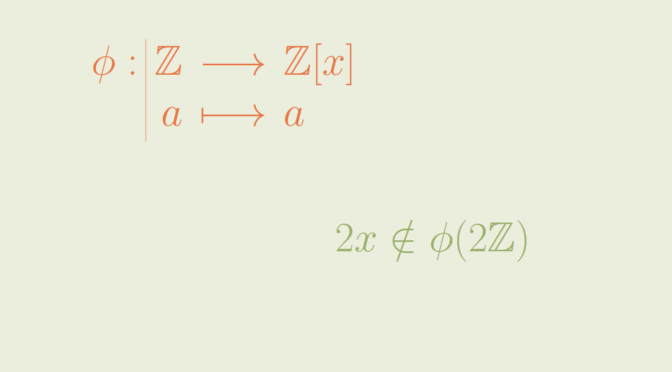# The image of an ideal may not be an ideal

If $$\phi : A \to B$$ is a ring homomorphism then the image of a subring $$S \subset A$$ is a subring $$\phi(A) \subset B$$. Is the image of an ideal under a ring homomorphism also an ideal? The answer is negative. Let’s provide a simple counterexample.

Let’s take $$A=\mathbb Z$$ the ring of the integers and for $$B$$ the ring of the polynomials with integer coefficients $$\mathbb Z[x]$$. The inclusion $$\phi : \mathbb Z \to \mathbb Z[x]$$ is a ring homorphism. The subset $$2 \mathbb Z \subset \mathbb Z$$ of even integers is an ideal. However $$2 \mathbb Z$$ is not an ideal of $$\mathbb Z[x]$$ as for example $$2x \notin 2\mathbb Z$$.